# ISEE Middle Level Math : How to find the perimeter of a parallelogram

## Example Questions

### Example Question #1 : Parallelograms

Possible Answers:

18 in

26 in

13 in

36 in

Correct answer:

26 in

Explanation:

To find the perimeter of a parallelogram, add the lengths of the sides. Opposite sides of a parallelogram are equivalent.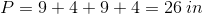### Example Question #2 : Parallelograms

If the perimter of a parallelogram isand one of the sides is, what is the other side length?

Possible Answers:The answer cannot be foundCorrect answer:Explanation:

The perimeter of a parallelogram is found by adding up all four sides.

Since there are two pairs of side with equal lengths, two sides must have a length of.

So the perimeter would be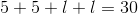or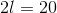.

To find the value of the other side length, you would divide the remaining perimeter not include in the other sides by.

So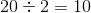.

That means the other side length would be.

### Example Question #3 : Parallelograms

If a parallelogram has side lengths ofand, what is the perimeter?

Possible Answers:Correct answer:Explanation:

The perimeter of a parallelogram is two times the two side lengths and add them together.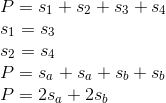Therefore, this particular problem becomes as follows.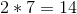and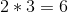so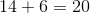.

### Example Question #1 : How To Find The Perimeter Of A Parallelogram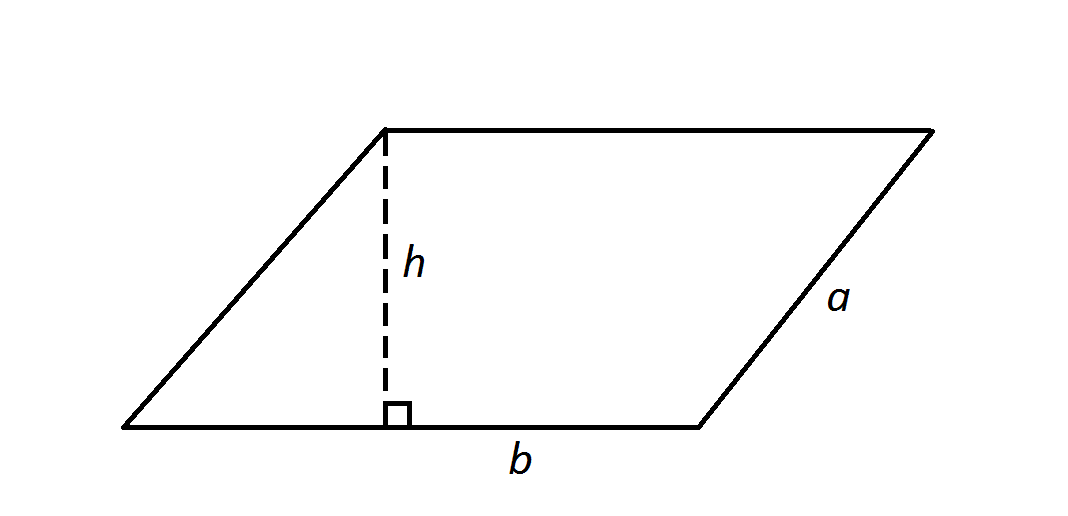Note: Figure NOT drawn to scale.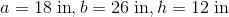, where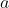and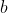represent side lengths of the parallelogram and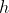represents the height.

Find the perimeter of the parallelogram in the diagram.

Possible Answers: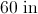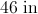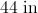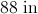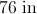Correct answer:Explanation:

The perimeter of the parallelogram is the sum of the four side lengths - here, that formula becomes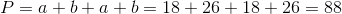.

Note that the heightis irrelevant to the answer.

### All ISEE Middle Level Math Resources